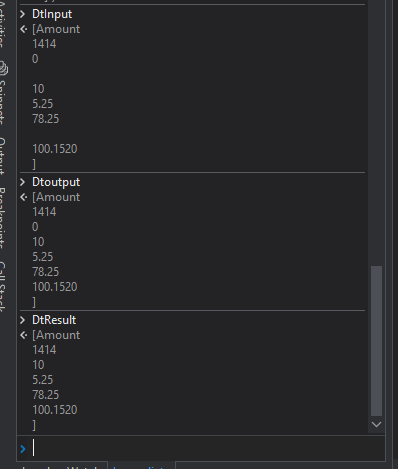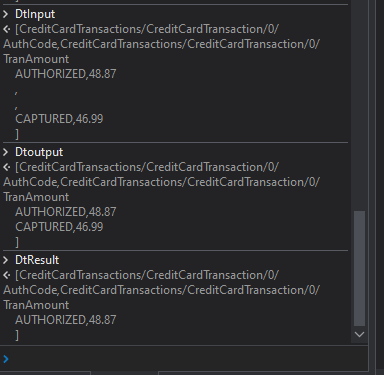# Filterdatatable convert string into Int

I have a datatable lets say Dt_Amount . This Dt_Amount has a column Amount .I need to filter Dt_Amount where Amount is greater than 0 .But here Amount is coming as string .How to filter Amount>0 in this in filter datatable.

@tharani.natarajan Cint(amount) that convert string to int in vb

Assign activity:
dtFiltered =

``````Dt_Amount.AsEnumerable().Where(Function (x) CInt(x("Amount").toString.Trim) > 0).CopyToDataTable
``````

Handling non valid values which are not parseable can be integrated when it is needed

Use this in assign

dt.AsEnumberable.Where(Function(x) CDBL(x(“Amount”).Tostring)>0).CopyToDatatable

cheers

Thanks ,however Amount in datatable is “0.00” .so getting error like conversion from string “” to type Integer is not valid

then its a double. we use CDBL method.

As int was mentioned above by the description, so we started with CInt

After having CDBL conversion from string “” to type double is not valid

The amount looks like “0.00” .double quotes in fronts and back of the value.

Amount =0.00

Hence it is not able convert it into int or double .how to eliminate double quotes from fronts and back of the value and then convert it to double.

After having CDBL conversion from string “” to type double is not valid

The amount looks like “0.00” .double quotes in fronts and back of the value.

Amount =0.00

Hence it is not able convert it into int or double .how to eliminate double quotes from fronts and back of the value and then convert it to double.

use like this

``````dt.AsEnumberable.Where(Function(x) CDBL(x(“Amount”).Tostring.Replace("""",""))>0).CopyToDatatable
``````

cheers

we mentioned by:

but you have to specify on how to handle non parseable amounts

• setting a default value e.g. 0
• filterout or keeping

still the same error@tharani.nataraj for each row in datatable
Assign currentrow(“Amount)=Cbdl(Currentrow (“Amount”).Replace(”“”, “”).Trim())
Then filter datatable using amount >0

1. Use Read range activity → Store it as `DtInput`

2. Use Filter Data Table activity → To remove the empty rows in the excel file and store the result in `DtOutput`

1. Use Assign activity and try with this expression

``````DtResult = Dtoutput.AsEnumerable().Where(Function (x) CDbl(x("Amount").toString.Trim) > 0).CopyToDataTable
``````

Check out this XAML file

FilterAmountGreaterThanZero.xaml (8.7 KB)

OutputRegards
Gokul

Maybe there are empty values in the data. How would you want to handle them? The below expression doesn’t include it in the Output (Assuming it is considered to be 0).

Could you check the below Expression :

``````Dt_Amount.AsEnumerable.Where(Function(x)x("Amount").ToString.IsNumeric andAlso CDbl(x("Amount").ToString)>0).CopyToDatatable
``````
1 Like

testing.xlsx (9.2 KB)

Attaching the excel for better understanding .I need check if value os column A is AUTHORIZED and Column B value is >0

Attaching the excel for better understanding .I need check if value os column A is AUTHORIZED and Column B value is >0
testing.xlsx (9.2 KB)

Use this

``````Dt_Amount.AsEnumerable.Where(Function(x) x("CreditCardTransactions/CreditCardTransaction/0/AuthCode").ToString.ToUpper.Contains("AUTHORIZED") andAlso CDbl(x("CreditCardTransactions/CreditCardTransaction/0/TranAmount").ToString)>0).CopyToDatatable
``````

cheers

Modifying the Above Expression to the requirement stated :

``````Dt_Amount.AsEnumerable.Where(Function(x)x(0).ToString.Equals("AUTHORIZED") andAlso (x(1).ToString.IsNumeric andAlso CDbl(x(1).ToString)>0)).CopyToDatatable
``````

Check if the above expression works. Let us know what is the output.
For Handling errors related to Direct CopyToDatatable, check the Tutorial post from @ppr above.

``````Dtoutput.AsEnumerable().Where(Function (x) CDbl(x(1).toString.Trim) > 0 And x(0).ToString.Contains("AUTHORIZED")).CopyToDataTable